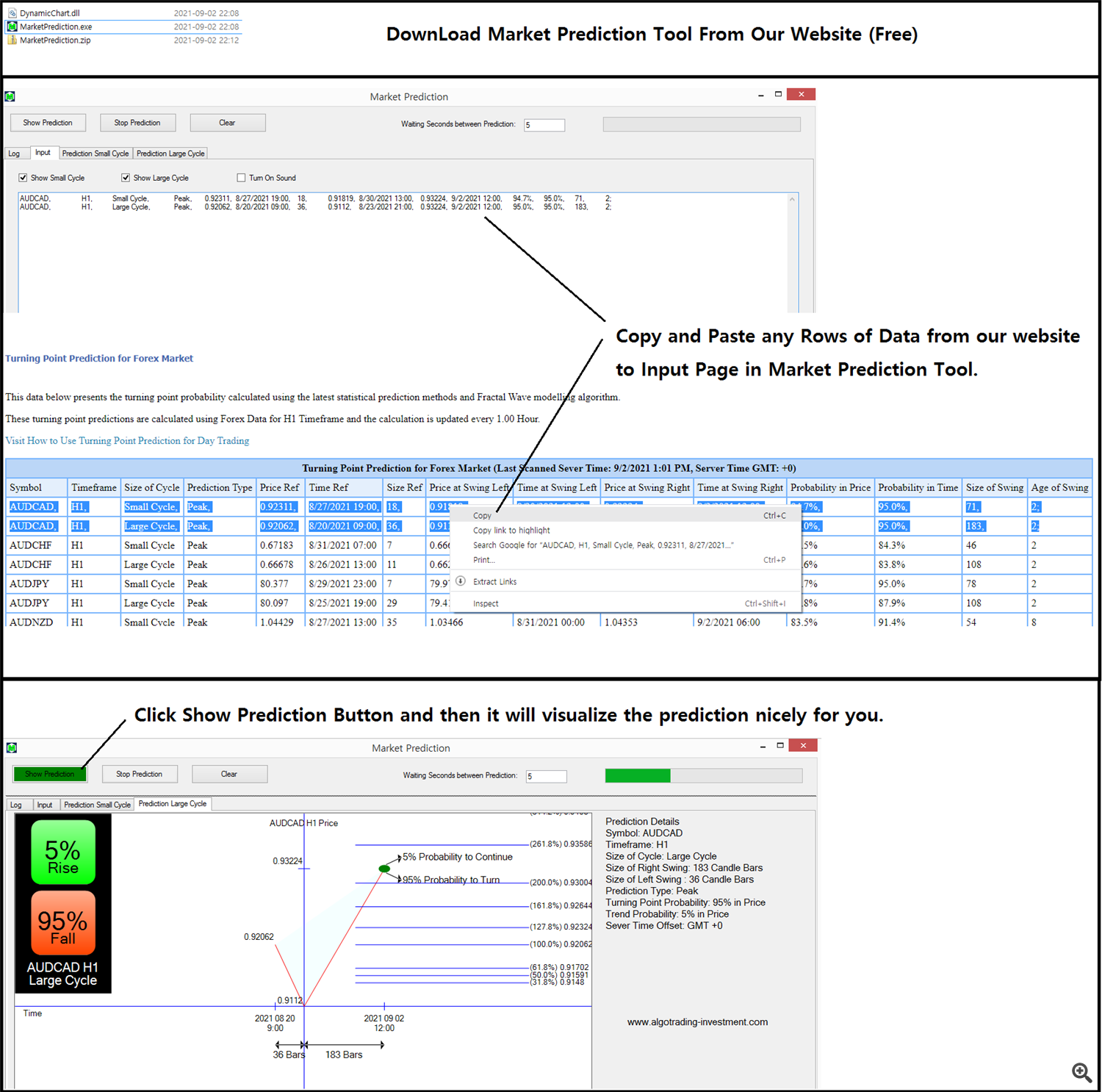# How To Use Forex Prediction With Fibonacci Analysis For Free – Trading Systems – 3 February 2022

In this article, we will explain how to use Forex Prediction with Fibonacci Analysis For Free in two steps. In the first step, you can access the Forex Prediction Webpage from the following link. You can book mark this Forex Prediction page below so you can access Forex Prediction any time.

From the above page, you will get access to the turning point prediction for Forex market including EURUSD, GBPUSD, USDJPY, BTCUSD, ETHUSD and so on. In fact, most of statistical predictions are not compatible with the actual trading strategy used by the real world trader. However, the turning point probability (and also the trend probability = 1 – turning point probability) is useful because the predictions were measured from the Fractal Wave. Remember that fractal wave is the direct representation of price action. For example, Support, Resistance, Fibonacci Ratio Analysis, Harmonic Pattern, Elliott Wave Theory, X3 Chart Pattern, Symmetric Triangle Pattern, Rising Wedge Pattern, Falling Wedge pattern, Double Top, Double Bottom, Channel, Renko Chart and so on are such technical analysis derived from Fractal Wave. You can consider the turning point probability as the quantified version of the price patterns. Hence, using the turning point probability with these price pattern is really beneficial in assessing the trend strength and risk for your trading. Especially, we recommend using the turning point probability together with any horizontal support resistance. For example, you can use the hand drawn support resistance, supply demand analysis, round number (=psychological number), pivot analysis, Fibonacci analysis, and so on. I am sure you can think of many horizontal support and resistance of your own.

In the second step, you can visualize the turning point probability and Fibonacci Analysis with this free Market Prediction tool from our website or from google drive. Anyway, you will get the same files.